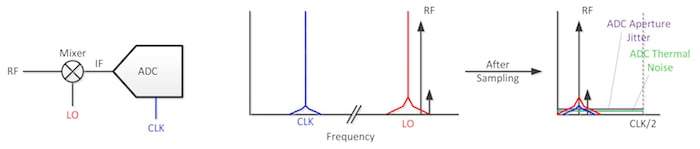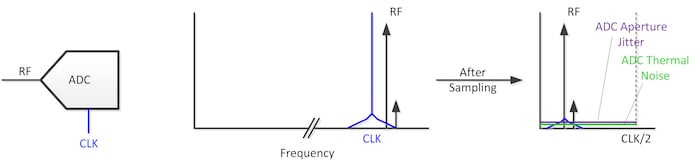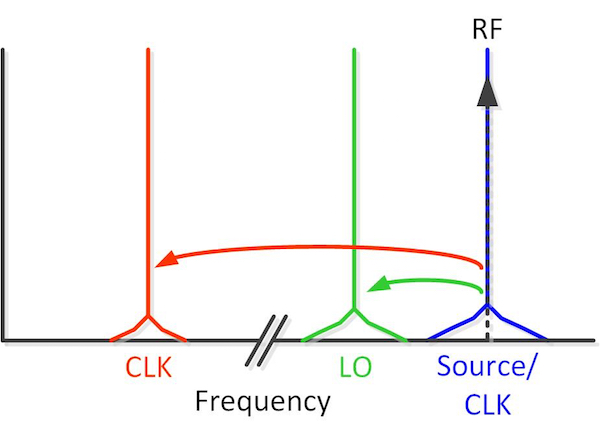Industry Article

# Clock-Noise Impact on RF Signals for Different Receiver Architectures

August 08, 2017 by Thomas Neu, Texas Instruments

## This article examines the common assumption that the clock-performance requirement for an RF sampling ADC is much more stringent compared to a heterodyne approach.

Recent advances in high-performance radio frequency (RF) data converters are enabling direct RF sampling up to 4GHz and beyond without significantly sacrificing performance.

The L-band (1-2GHz) and S-band (2-4GHz) are traditionally used either as direct input or as an intermediate frequency (IF) when downconverting from a higher RF frequency (like in a Ku-band radar, for example). Rather than designing a heterodyne receiver to sample these bands at a lower frequency, system designers are eager to simplify their receiver signal chain using a direct RF sampling analog-to-digital converter (ADC), resulting in reduced size, weight and power.

Clock phase-noise performance is a key parameter to overall receiver performance – both for a traditional heterodyne receiver as well as for a direct RF sampling implementation. During a blocking condition with an in-band interferer, the clock phase-noise performance determines the minimum detectable signal power. In radar systems, the receiver close-in phase-noise performance directly impacts the accuracy of the speed and direction of the target.

Since the data converter sampling clock is such a critical element in a direct RF receiver (and I’m often asked about it), in this article I’ll examine the common assumption that the clock-performance requirement for an RF sampling ADC is much more stringent compared to a heterodyne approach.

A traditional downconversion (heterodyne) receiver employs a mixer together with a local oscillator (LO) to frequency shift the RF input signal to a lower IF, which the data converter digitizes (see Figure 1).##### Figure 1. Heterodyne receiver approach.

The mixing operation is a convolution of the LO and the RF input in the frequency domain (multiplication in the time domain). As a result of mixing, the phase noise from the LO gets added to the input signal and increases the overall noise floor. This is typically referred to as reciprocal mixing.

During the ADC sampling process, the clock phase noise, which includes ADC aperture jitter and external clock phase noise, gets added to the IF input signal as well. However, the clock phase-noise amplitude scales based on the relationship between the input and the clock frequency $$\text{20Log}\left( \frac{\text{IF}}{\text{CLK}} \right)$$. Additionally, you need to consider the ADC thermal noise needs as well.

Equation 1 calculates the resulting phase-noise amplitude of the sampled IF signal:

$$\text{P}{{\text{N}}_{\text{IF}}}\text{=20Log}\left( \sqrt{\text{PN}_{\text{LO}}^{\text{2}}\text{+PN}_{\text{ADC }\!\!\_\!\!\text{ PN}}^{\text{2}}\text{+PN}_{\text{ADC }\!\!\_\!\!\text{ THERMAL}}^{\text{2}}} \right)$$

##### Equation 1

where $$[\text{P}{{\text{N}}_{\text{ADC }\!\!\_\!\!\text{ PN}}}\text{=P}{{\text{N}}_{\text{CLK}}}\text{ }\!\!\times\!\!\text{ 20LOG }\!\!~\!\!\text{ }\left( \frac{\text{IF}}{\text{CLK}} \right)$$​ and PNCLK is the combination of external clock phase noise and internal ADC aperture jitter (phase noise). Both components experience the amplitude scaling of 20LOG[IF/CLK].

In a modern receiver architecture, the RF sampling ADC digitizes the input signal directly at RF without the use of a downconversion stage, as illustrated in Figure 2. Removing a downconversion stage can significantly simplify the receiver signal chain and save power, cost, and printed circuit board (PCB) area.##### Figure 2. Direct RF sampling approach.

As shown earlier, the ADC aperture jitter and clock phase-noise amplitudes first scale by $$\text{P}{{\text{N}}_{\text{CLK}}}$$ before adding to the input signal together with the ADC thermal noise (Equation 2):

$$\text{P}{{\text{N}}_{\text{RF}}}\text{=20Log}\left( \sqrt{\text{PN}_{\text{ADC }\!\!\_\!\!\text{ PN}}^{\text{2}}\text{+PN}_{\text{ADC }\!\!\_\!\!\text{ Thermal}}^{\text{2}}} \right)$$

##### Equation 2

where $$\text{P}{{\text{N}}_{\text{ADC }\!\!\_\!\!\text{ PN}}}\text{= P}{{\text{N}}_{\text{CLK}}}\text{ }\!\!\times\!\!\text{ 20Log}\left( \frac{\text{RF}}{\text{CLK}} \right)$$ with PNCLK again the combination of external clock phase noise and internal ADC aperture jitter (phase noise).

Assuming equivalent thermal noise (in decibels relative to full scale/hertz) for both IF and RF sampling data converters, the receiver noise degradation ultimately comes down to LO and clock phase noise.

In order to better compare the clock phase-noise requirements for the two receiver architectures, you can normalize the phase noise of the frequency sources (LO, ADC CLK) by deriving them from the same source, as shown in Figure 3. For example, an LO at half the frequency of the source would have 6dB lower phase noise than the source itself. In order to keep things simple, I picked the same RF frequency for the frequency source.### Normalizing the Clock Phase Noise Requirement for the Downconversion Receiver

Equation 3 calculates the frequency of the local oscillator (LO) as:

$$\text{LO=RF-IF=RF }\!\!\times\!\!\text{ (1-a)}$$

##### Equation 3

where $$\text{IF=a }\!\!\times\!\!\text{ RF}$$.

Assuming low-side injection of the mixer, Equation 4 calculates the phase noise of the LO from a common clock source at RF as:

$$\text{P}{{\text{N}}_{\text{LO}}}\text{=P}{{\text{N}}_{\text{RF}}}\text{ }\!\!\times\!\!\text{ 20Log}\left( \frac{\text{LO}}{\text{RF}} \right)\text{=P}{{\text{N}}_{\text{RF}}}\text{ }\!\!\times\!\!\text{ 20Log}\left( \text{1-a} \right)$$

##### Equation 4

Equation 5 calculates the phase noise of the sampled ADC output as a function of the clock phase noise as:

$$\text{P}{{\text{N}}_{\text{ADC}}}\text{=P}{{\text{N}}_{\text{CLK}}}\text{ }\!\!\times\!\!\text{ 20Log}\left( \frac{\text{IF}}{\text{CLK}} \right)$$

##### Equation 5

You can derive the phase noise of the external ADC sampling clock from the common RF clock source with Equations 6 and 7 (not including aperture jitter for simplicity):

$$\text{P}{{\text{N}}_{\text{CLK}}}\text{=P}{{\text{N}}_{\text{RF}}}\times \text{20Log}\left( \frac{\text{CLK}}{\text{RF}} \right)$$

##### Equation 6

$$\text{P}{{\text{N}}_{\text{ADC}}}\text{=P}{{\text{N}}_{\text{RF}}}\times 2\text{0Log}\left( \frac{\text{IF}}{\text{CLK}} \right)\times \text{20Log}\left( \frac{\text{CLK}}{\text{RF}} \right)\text{=P}{{\text{N}}_{\text{RF}}}\times \text{20Log}\left( \frac{\text{IF}}{\text{RF}} \right)\text{=P}{{\text{N}}_{\text{RF}}}\text{ }\!\!~\!\!\text{ }\times \text{20Log}\left( \text{a} \right)$$

##### Equation 7

Combine the LO and ADC phase-noise contributions using Equation 8:

\begin{align}& \text{P}{{\text{N}}_{\text{total}}}\text{=20Log}\left( \sqrt{\text{PN}_{\text{LO}}^{\text{2}}\text{+PN}_{\text{ADC}}^{\text{2}}} \right) \\ & \text{=20Log}\left( \sqrt{{{\left( \text{P}{{\text{N}}_{\text{RF}}}\times \left( \text{1-a}\right) \right)}^{\text{2}}}\text{+}{{\left( \text{P}{{\text{N}}_{\text{RF}}}\times \text{a}) \right)}^{\text{2}}}} \right)\\ & \text{=P}{{\text{N}}_{\text{RF}}}\text{ }\!\!~\!\!\text{ }\times \text{10log}\left( {{\left( \text{1-a} \right)}^{\text{2}}}\text{+}{{\text{a}}^{\text{2}}} \right) \end{align}

##### Equation 8

Where $$\text{a=}\frac{\text{IF}}{\text{RF}}$$.

This shows that the amplitude of the phase noise, which gets added to the input signal, is dependent on the IF frequency.

Assuming that the IF is ~10% of the RF frequency (for example, if IF = 180MHz, RF = 1.8GHz), the phase-noise contribution of the LO normalized to the RF frequency changes by 20LOG(0.9) = -0.91dB. This means that the LO phase noise improves by 0.91dB. The clock phase-noise contribution of the ADC changes by 20LOG(a = 10%) = -20dB. As a result, the added phase noise of the sampled signal is about 0.86dB lower than the phase noise of the RF clock source.

The ADC aperture jitter, assuming a similar level as the external clock phase noise, would have only a minor impact on the overall result.

### Normalizing the Clock Phase Noise Requirement for the RF ADC

Equation 9 calculates the phase noise of the sampled ADC output as a function of the clock phase noise:

$$\text{P}{{\text{N}}_{\text{ADC}}}\text{=P}{{\text{N}}_{\text{CLK}}}\text{ }\!\text{ }\!\times\!\text{ }\!\text{ 20log}\left( \frac{\text{RF}}{\text{CLK}} \right)$$

##### Equation 9

You can derive the phase noise of the ADC clock from the common RF clock source using Equations 10 and 11:

$$\text{P}{{\text{N}}_{\text{CLK}}}\text{=P}{{\text{N}}_{\text{RF}}}\text{ }\!\text{ }\!\times\!\text{ }\!\text{ 20log}\left( \frac{\text{CLK}}{\text{RF}} \right)$$

##### Equation 10

$$P{{N}_{ADC}}=P{{N}_{RF}}~\times ~20log\left( \frac{RF}{CLK} \right)\times ~20log\left( \frac{CLK}{RF} \right)=P{{N}_{RF}}$$

##### Equation 11

When normalized to the RF frequency, there is no change in amplitude at all. The additive phase noise of the RF ADC is the same as the phase noise of the RF clock source. Unlike the heterodyne receiver, the aperture jitter of an RF ADC can cause significant phase-noise degradation.

The heterodyne receiver shows a small improvement, which is dependent on the IF frequency. The larger the IF frequency (in percentage of the RF frequency), the larger the difference in the normalized phase-noise requirements for the two cases.

Thus, the two architectures in fact show very minor external clock phase-noise requirements. A typical use case with IF = 0.1 x RF shows only about a 0.9dB normalized phase-noise relaxation compared to the direct RF sampling approach. So the clock-noise requirements for both architectures are pretty comparable. The major difference is the internal ADC aperture jitter, which becomes more dominant as the RF input frequency increases.

Industry Articles are a form of content that allows industry partners to share useful news, messages, and technology with All About Circuits readers in a way editorial content is not well suited to. All Industry Articles are subject to strict editorial guidelines with the intention of offering readers useful news, technical expertise, or stories. The viewpoints and opinions expressed in Industry Articles are those of the partner and not necessarily those of All About Circuits or its writers.